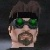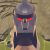## Welcome to the Second Life Forums Archive

These forums are CLOSED. Please visit the new forums HERE

# LSLisp v.2

Jarod Godel
UtilitarianJoin date: 6 Nov 2003
Posts: 729
02-11-2004 09:24
This is a miniature Lisp parser written in LSL. I do not consider it an implementation of Lisp since it does not handle many things like lists or recursion. It is a parser, though, as well as a basic calculator. Hopefully there will be a version 3 someday, but until then, for anyone who is intersted in creating a parser in LSL, here you go...

This "script" requires two prims. A parent prim, named LSLisp, and a child prim, called Lisprim, that goes inside the first's inventory. (There's a brief explanation of how it all fits together in this post.) The first two scripts, "rezer" and "namer" go inside LSLisp. The third, "lispy," goes inside Lisprim.

Here's rezer:

CODE
`// rezer// This program listens for a Lisp line, counts the parenthesis in it,// rezes an equal number of Lisp parsers, and broadcasts the equation// off to the first.// Global variablesinteger BCHAN = 20000;string PROCNAME = "Lisprim";string OWNER;// Counts the parenthesesparser(string code){    integer opc = 0;    integer cpc = 0;    integer cntr = 0;        while (llGetSubString(code, cntr, cntr))    {        // counts opening parenthesis        if (llGetSubString(code, cntr, cntr) == "(")        {            opc++;        }                // counts closing parenthesis        if (llGetSubString(code, cntr, cntr) == ")")        {            cpc++;        }                cntr++;    }                        // If cpc and opc are equal    if (cpc == opc && cpc > 0)    {        integer rc = 0;     // rez counter        float ht = .5;      // height        float hc = .5;                while (rc < cpc)        {            integer rd = 0; // rez delay                        llRezObject(PROCNAME, llGetPos() + <0,0,hc>, <0,0,0>, <0,0,0,0>, 1);            hc = hc + ht;            rc++;                        while (rd < 10)            {                rd++;            }        }        llSay(BCHAN + opc, code);    }        // If not    else    {        llSay(0, "There was a missing parenthesis.");    }}// Main routinedefault{    state_entry()    {        OWNER = llKey2Name(llGetOwner());        llSay(0, "LSLisp v.2");                state ListenState;    }}// Listen Statestate ListenState{    state_entry()    {        llListen(0, OWNER, NULL_KEY, "");    }        listen(integer channel, string name, key id, string message)    {        parser(message);    }}`

Here's namer:

CODE
`// namer// This program listens on a certain channel for Lisp parsers,// and when they ask it for a channel number it broadcasts it// out to them so they can listen accordingly.// Global variablesinteger BCHAN = 20000;integer TEMP;integer current = BCHAN;string NAME = "Lisprim";// Main routinedefault{    on_rez(integer param)    {        llResetScript();    }        state_entry()    {        llListen(BCHAN, NAME, NULL_KEY, "");    }        listen(integer channel, string name, key id, string message)    {        // initializing        if (message == "on")        {            current += 1;            llSay(BCHAN, (string)current);        }                      // denitializing        else if (message == "off")        {            current--;        }                // reset        else if (message == "reset")        {            current = BCHAN;        }    }}`

Here's lispy:

CODE
`// lispy// This is the program that parses a section of Lisp code// Global variablesinteger LISTME;integer LISTMETOO;integer BCHAN = 20000;integer CHAN = BCHAN;string MAIN = "LSLisp";string gotvar;// Functionsstring lispfuncs(string block){    list words = llParseString2List(block, [" "], [" "]);    string cmd = llList2String(words, 0);    string retval;    integer numcnt = 1;    integer buffer = 0;    integer sort = TRUE;    // adds    if (cmd == "+" || cmd == "add")    {        while (llList2String(words, numcnt) != "")        {            buffer = buffer + (integer)llList2String(words, numcnt);            numcnt++;        }    }        // subtracts    else if (cmd == "-" || cmd == "subtract")    {        buffer = (integer)llList2String(words, numcnt);        numcnt++;        while (llList2String(words, numcnt) != "")        {            buffer = buffer - (integer)llList2String(words, numcnt);            numcnt++;        }    }        // multiply    else if (cmd == "*" || cmd == "multiply")    {        buffer = (integer)llList2String(words, numcnt);        numcnt++;        while (llList2String(words, numcnt) != "")        {            buffer = buffer * (integer)llList2String(words, numcnt);            numcnt++;        }    }        // square    else if (cmd == "sqr" || cmd == "square")    {        buffer = (integer)llList2String(words, numcnt);        buffer = buffer * buffer;    }        // Resets namer    else if (cmd == "reset")    {        llSay(BCHAN, "reset");        llSay(0, "Reset");        llDie();    }        // greater    else if (cmd == ">")    {        if ((integer)llList2String(words, 1) > (integer)llList2String(words, 2))        {            buffer = 1;        }                else if ((integer)llList2String(words, 1) < (integer)llList2String(words, 2))        {            buffer = 0;        }    }        // lesser    else if (cmd == "<")    {        if ((integer)llList2String(words, 1) < (integer)llList2String(words, 2))        {            buffer = 1;        }                else if ((integer)llList2String(words, 1) > (integer)llList2String(words, 2))        {            buffer = 0;        }    }        // equal    else if (cmd == "=")    {        if ((integer)llList2String(words, 1) == (integer)llList2String(words, 2))        {            buffer = 1;        }                else        {            buffer = 0;        }    }    // Returns the value        if (sort == TRUE)    {        return (string)buffer;    }    else    {        return retval;    }   }// Parserlispparser(string line){    // Local variables    list chars;    integer x = 0;    // parses the string to a list        while (llGetSubString(line, x, x) != "")    {        chars = chars + llGetSubString(line, x, x);        x++;    }    integer y = 0;    integer fp = 0;    integer lp = 0;    string templine = "";        // resets x    x = 0;    // Finds the opening parenthesis        while (llGetSubString(line, x, x) != "")    {        if (llList2String(chars, x) == "(")        {            fp = x;            y = x;        }        x++;    }    // resets x    x = 0;    // finds the first closing parenthesis after the last opening one        while (x == 0)    {        if (llGetSubString(line, y, y) == ")")        {            lp = y;            x = 1;        }        y++;    }        // assigns the returned value to a variable    string worked = lispfuncs((string)llList2List(chars, fp + 1, lp - 1));    // resets x    x = 0;            // rebuilds the first part of the string    if (fp > 0)    {        while (x < fp)        {            templine = templine + llGetSubString(line, x, x);            x++;        }    }            // adds the returned value    templine = templine + worked;    if (llGetSubString(line, lp, lp) == ")")    {        x = lp + 1;    }    // adds the rest of the line    while (llGetSubString(line, x, x) != "")    {        templine = templine + llGetSubString(line, x, x);        x++;    }    // If the first character is not a parenthesis    if (llGetSubString(templine, 0, 0) != "(")    {        llSay(0, templine);    }        // If it is    else    {        llSay(CHAN - 1, templine);    }        llSay(BCHAN, "off");    llDie();}// Main routinedefault{    on_rez(integer param)    {        llResetScript();    }        state_entry()    {        llSay(CHAN, "on");        LISTME = llListen(CHAN, MAIN, NULL_KEY, "");    }        listen(integer channel, string name, key id, string message)    {        if (message != "")        {            if (channel == 20000)            {                CHAN = (integer)message;                llListenRemove(LISTME);                LISTME = llListen(CHAN, "", NULL_KEY, "");            }                    else if (channel == 19999)            {                gotvar = message;                llListenRemove(LISTME);                            LISTME = llListen(CHAN, "", NULL_KEY, "");            }                    else            {                lispparser(message);            }        }    }}`

If this code ever locks up, resetting "namer" will usually fix the problem. Tighter listening is planned for the next version.
_____________________
"All designers in SL need to be aware of the fact that there are now quite simple methods of complete texture theft in SL that are impossible to stop..." - Cristiano Midnight

Ad aspera per intelligentem prohibitus.
Huns Valen
Don't PM me here.Join date: 3 May 2003
Posts: 2,749
04-11-2004 03:44
I'm sorry if I missed it, but can you post a sample LISP script to go with this?
_____________________
Jarod Godel
UtilitarianJoin date: 6 Nov 2003
Posts: 729
04-12-2004 15:10
(+ 1 (+ 2 (+ 3 (+ 4 5))))

Is that what you meant? It's not a full implementation, and probably not much more than a calculator my most standards.
_____________________
"All designers in SL need to be aware of the fact that there are now quite simple methods of complete texture theft in SL that are impossible to stop..." - Cristiano Midnight

Ad aspera per intelligentem prohibitus.